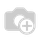• Fiyatlar

# how to display different wizard view for one2many field than the original view?

I'm using a module for Odoo documents management, in the view of the main directory there is an one2many field for sub-directories and it point's to the same model so while creating it's open a wizard exactly like the form which it locates, I need to hide some fields form this wizard and add some new fields related to sub-directories only, how can I do that, this the code

```` class Directory(models.Model):````
````_name = 'muk_dms.directory'``_description = "Directory"````
````_inherit = [``    'muk_utils.mixins.hierarchy',``    'muk_security.mixins.access_rights',``    'muk_dms.mixins.thumbnail',``]````
````_order = "name asc"````
````_parent_store = True``_parent_name = "parent_directory"````
````_parent_path_sudo = False``_parent_path_store = False````
````_name_path_context = "dms_directory_show_path"````
````#----------------------------------------------------------``# Database``#----------------------------------------------------------````
````name = fields.Char(````    string="Name",
required````    required=True,``    index=True)````
````is_root_directory = fields.Boolean(````    string="Is Root Directory",
default````    default=False,````    help="""Indicates if the directory is a root directory. A root directory has a settings object,
while a directory with a set parent inherits the settings form its parent."""````        while a directory with a set parent inherits the settings form its parent.""")````
````root_storage = fields.Many2one(````    comodel_name='muk_dms.storage',
string````    string="Root Storage",``    ondelete='restrict')````
````storage = fields.Many2one(``    compute='_compute_storage',````    comodel_name='muk_dms.storage',
string````    string="Storage",``    ondelete='restrict',``    auto_join=True,``    readonly=True,``    store=True)````
````parent_directory = fields.Many2one(````    comodel_name='muk_dms.directory',
domain````    domain="""[('permission_create', '=', True)]""",``    context="{'dms_directory_show_path': True}",``    string="Parent Directory",``    ondelete='restrict',``    auto_join=True,``    index=True)````

as it shown in above code the last field is get the data form the same model, how can i display wizard with different fields Thanks in advance,

1 Yorum